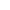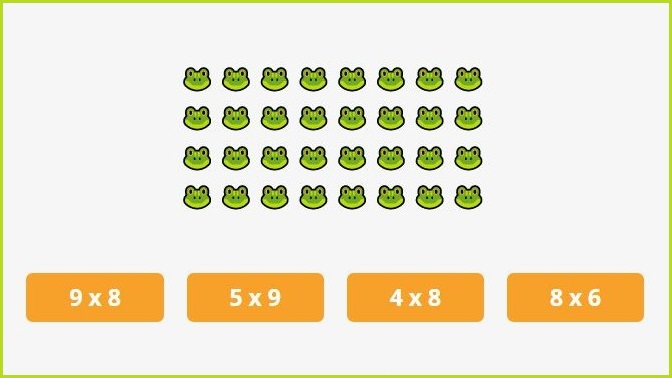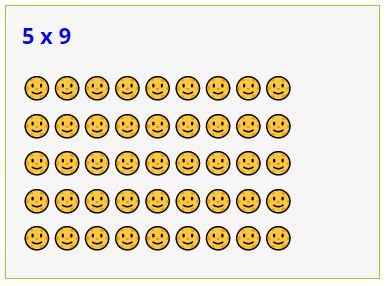﻿ Multiplication Arrays Games ONLINE + Worksheets

Page No. 1056

# Multiplication Arrays Games

Multiplication Array Games ONLINE. Using arrays with multiplication. Learn what are multiplication arrays and how to teach multiplication using arrays. Free printable multiplication arrays worksheets PDF. Rectangular arrays multiplication. multiplication array practice. teaching multiplication with arrays. multiplication facts using arrays. array model multiplication. using arrays to teach multiplication. array multiplication games.

What problem does this array represent?

New game## Multiplication arrays games ONLINEWhat problem does this multiplication array represent? Click on the correct answer. Press the button "New game" and play another multiplication array game.

## Free printable multiplication arrays worksheets PDF

Download free printable multiplication arrays worksheets in PDF. Click the image bellow and generate free printable multiplication using arrays worksheets.

You can generate as many different multiplication arrays worksheets as you want. Find more free multiplication worksheets here.

## What are multiplication arrays

Multiplication arrays are a visual representation of multiplication that use a rectangular grid of dots or objects to show the relationship between the factors and the product. An array is a way to show multiplication as rows and columns.

Multiplication array is created by drawing a rectangle that is divided into rows and columns. Each cell in the rectangle represents a single unit, and the total number of cells represents the product of the two factors being multiplied.

#### Printable multiplication games

50 multiplication puzzles

Fun printable single digit multiplication games. 50 different pictures, many one digit multiplication tasks.

US\$ 3.00

## Multiplication arrays game

We use multiplication arrays to illustrate single digit multiplication facts, it is very helpful especially for younger children.

### Multiplication arrays

For example, an array can be used to show the multiplication of 5 and 9.

This array has 5 rows and 9 columns, and each picture represents a single unit. By counting the number of pictures, we can see that the product of 5 and 9 is 45: this means 5 x 9 = 45.## Using arrays in multiplication

Multiplication arrays are a useful tool for visual learners and can be used to demonstrate multiplication in a concrete way. You can play this online multiplication game on any device with an internet connection.

They can also be used to help learners understand the concept of multiplication as repeated addition. For example, the array for 3 x 4 can be thought of as 3 groups of 4, or as 4 groups of 3, each containing one picture.

## Teaching multiplication using arrays

Teaching multiplication using arrays is one of effective ways to help students understand the concept of multiplication.

Together with multiplication flash cards this game helps as well to memorize multiplication facts.### Here are some steps to teach multiplication using arrays

1. Introduce the concept of multiplication: 👉 Begin by explaining what multiplication is and why it is useful. You can explain that multiplication is a way of representing repeated addition, and that arrays are one way to visualize multiplication. Watch it on YouTube here.
2. Show examples of multiplication arrays: 👉 Draw a few arrays on the board and ask students to count the number of dots in each array. Make sure to use a variety of array sizes and multiplication facts to help students see the patterns.
3. Have students create their own arrays: 👉 Give students a multiplication fact, such as 4 x 3, and ask them to draw an array to represent the fact. Encourage students to use dots or objects to create the array and to label the rows and columns with the factors.
4. Practice multiplication with arrays: 👉 Once students are comfortable creating arrays, provide them with multiplication problems to solve using arrays. For example, you could ask students to find the product of 5 x 2 using an array. Have them draw the array and count the dots to find the answer.
5. Reinforce learning: 👉 Use arrays in other activities to reinforce learning. For example, you could create a game where students take turns drawing arrays and guessing the multiplication fact. Or, you could ask students to create arrays using different materials, such as counters or beans.

### Online multiplication arrays games

Incorporate this interactive multiplication arrays game into your lesson plan. It provides additional practice and develop a deeper understanding of the relationship between factors and products.